## Introduction

The advent of new material properties has led to the development of new applications in electromagnetism. For example, metamaterials1,2,3, composed of sub-wavelength resonant structures, readily enable us to use negative- or zero-refractive indices2 as well as artificially engineered high impedance surfaces3 which allow surface wave properties to be controlled. These unusual properties were applied to the development of a diffraction-limitless lens4,5, cloaking device6,7,8, unusually thin absorbers (<λ/4 where λ is the wavelength of the incoming wave)9,10,11, etc. Importantly, such new electromagnetic properties and applications were widely exploited in other disciplines such as acoustics12,13,14,15, thermodynamics16 and vibration engineering17,18. Moreover, a recent study on metasurface absorbers containing circuit elements such as diodes and capacitors demonstrated a new property called waveform dependence19,20. Interestingly these metasurfaces absorbed only short sine wave pulses, while transmitting continuous waves (CWs) even at the same frequency. In this study we present a new concept of metasurfaces to fully control waveforms, i.e. selective absorption of either short pulses or CWs as well as absorption or transmission of specific waveforms in between. This new property termed waveform selectivity allows us to distinguish incoming waves in an unusual manner depending not only on the frequency but also on the waveform. Thus the waveform selectivity has a potential to develop new kinds of techniques and applications in electromagnetism as well as in other disciplines.

The waveform selectivity is made possible by integrating the rectification of microwave diodes with the time-domain responses of capacitors and inductors (Figs. 1(a) and 1(b)). First, a diode converts the frequency of an incoming signal to an infinite set of frequency components, but most of the energy is at zero frequency10. The conversion to zero frequency is further enhanced if a four-diode bridge is used19 (see Supplementary Information for details). Also, a capacitor has an impedance calculated from 1/jωC, where j2 = −1, ω is the angular frequency (ω = 2πf and f is the frequency) and C is the capacitance. Since the rectified signal contains the zero frequency component, the capacitor stores the energy but is gradually charged up. This indicates that capacitors allow current to come in during an initial time period. On the other hand, an inductor has impedance of jωL, where L is the inductance and generates an electromotive force opposing the incoming current. This force, however, is gradually weakened due to the zero frequency component and more current comes in. Thus, inductors accept incoming current after an initial time period.

In addition, integration of these time domain responses with a resistor leads to effective absorption of either short or long waveforms. Fig. 1(c) illustrates such circuit configurations deployed between square patches of metasurfaces. In this figure a capacitor is connected to a resistor in parallel, or an inductor in series. Incoming waves have electric field normal to the metasurfaces, which charges the edge of each patch either positively or negatively and thus generates strong electric field across the gaps (this electric field turns on the diodes). Under these circumstances the capacitor is capable of fully storing the energy of a short pulse during the illumination and discharges it into the resistor, which dissipates the energy before a next short pulse comes in. For a CW, however, the capacitor is fully charged up. As a result, the incoming wave transmits over the metasurface. Regarding the inductor, a short pulse cannot be rectified by diodes due to the presence of the electromotive force, resulting in no energy dissipation in the series resistor. However, reduction of the force due to long-term illumination permits the rectification, leading to energy dissipation in the resistor. For these reasons a short pulse or CW is expected to be absorbed by the combination of a resistor with a capacitor or inductor, respectively.

Importantly, selective absorption and transmission demonstrated below are not due to the variation in the bandwidth of the incoming frequency, since the bandwidth of the signals is small compared to that of the surface without any nonlinear elements (e.g. provided that frequency and pulse width are respectively 4.0 GHz and 50 ns as set below, the one cycle is 0.25 ns and corresponds to only a 200th of the pulse duration).

On the basis of this theory, a metasurface was designed and built up as in Figs. 1(d) and 1(e) in order to absorb incoming surface waves. The simulation model had nine periodic unit cells along the propagation direction of a surface wave on the bottom of a TEM (transverse electromagnetic) waveguide. The measurement sample was fabricated under the same circumstances but with a few differences. For example, since the measurement required to use TE (transverse electric) waveguides (WR284 for up to 3.95 GHz and WR187 beyond 3.95 GHz) as realistic waveguides, the measurement sample had several unit cells along not only the direction of the wave propagation (k in Fig. 1(e)) but also that of the incident magnetic field (H) to fully occupy the bottoms of the waveguides. In addition, the measurement used commercial schottky diodes (Avago; High Frequency Detector Diodes HSMS-2863/2864), while the simulation used a SPICE model. The capacitors and inductors respectively had capacitance C = 1 nF and inductance L = 100 μH, while the resistors used with the capacitors and inductors respectively had resistances Rc = 10 kΩ and Rl = 5.5 Ω, where the measurement sample of the inductor-based metasurface did not use resistor chips, since the inductor chips already contained some resistance value, which was the same as Rl. The self-resonant frequencies of the capacitor and inductor chips, fc and fl, were respectively 300 and 10 MHz. Note that the time constants of the capacitor-based and inductor-based metasurfaces, determined by RcC and L/Rl, were respectively 10 μs and ~18 μs. More details on the simulation and measurement are described in Fig. 1 and Supplementary Information.

## Results

First of all, a capacitor-based metasurface and inductor-based metasurface were numerically tested with 15 dBm signals at different frequencies as shown in Figs. 2(a) and 2(d), respectively. As a result, the capacitor-based and inductor-based metasurfaces respectively absorbed short pulses (50 ns long) and CWs more effectively. Next, the frequency was fixed at 4.2 GHz, where either a short pulse or CW was strongly absorbed, while the other was weakly absorbed. Under this circumstance, the capacitor-based metasurface demonstrated a clear transition between the short pulse and CW as plotted by the closed squares of Fig. 2(b)19. This is because the capacitors used were gradually charged up, as the pulse width increased. The measurement was performed under the same circumstances except the input frequency set to 4.0 GHz due to a minor shift of the entire feature to a lower frequency region (see Fig. 11 of Supplementary Information). As plotted by the open squares of Fig. 2(b), the measurement result also demonstrated that the absorbing performance decreased, as the pulse width increased. On the other hand, the inductor-based metasurface gradually enhanced the absorbing performance by increasing the pulse width as Fig. 2(e), since the electromotive force was weakened and the incoming rectified energy was dissipated with the series resistors. The reasons for the differences between these simulated and measured results are explained by circuit parasitics (e.g. extra capacitances in diodes) and superimposed direct current characteristics. The former reason increased the time constant of the capacitor-based metasurface, while the latter decreased that of the inductor-based metasurface. The time domain responses of these two waveform-dependent metasurfaces to 15 dBm CWs are seen in Figs. 2(c) and 2(f), respectively. As expected from Figs. 2(b) and 2(e), the capacitor-based (inductor-based) metasurface gradually increased (decreased) the transmitted power. These plots also show that the reected powers were limited.

Such a waveform dependence can be more exibly designed by combining each of the circuit configurations with another. The insets of Figs. 3(a) and 3(d) illustrate circuit configurations containing the two types of circuit elements either in parallel or in series. For example, previously the individual capacitor-based metasurface absorbed short pulses but transmitted long pulses, which can be now absorbed by the parallel inductor part as demonstrated in Fig. 3(a). Interestingly, however, such a metasurface can still transmit some waveforms that were weakly absorbed by both of the individual metasurfaces (the closed squares of Fig. 3(b)). In this case, the absorptance value at each pulse width is close to the larger value of the individual structures (cf. Figs. 2(b) and 2(e)). Besides, as plotted by the closed circles of Fig. 3(b), the variation of the absorptance curve can be controlled by varying the time constants RcC and L/Rl (now C = 100 pF, L = 1 mH, Rc = 10 kΩ, Rl = 31.2 Ω, fc = 1.02 GHz and fl = 2.4 MHz), since these parameters determine the saturation of each curve. This was experimentally demonstrated by the open circles of Fig. 3(b), where an incoming wave was more transmitted when the pulse width was around 0.5 μs. The differences between the measured and simulated results were mainly due to some circuit parasitics and the superimposed direct current characteristics as explained above. Such behaviour of the parallel-type metasurface (the closed squares of Fig. 3(b)) can also be understood from Fig. 3(c), which reveals temporal enhancement of transmitted power.

In contrast, the series-type metasurface drawn by the inset of Fig. 3(d) can selectively absorb specific waveforms. Again, in Fig. 2 the individual capacitor-based metasurface temporarily stored the incoming energy in capacitors to dissipate it in resistors. However, this is now prevented by the electromotive force of the inductors. Likewise, long-pulse current cannot be absorbed by the inductor part, as the capacitors are fully charged up before the current reaches the inductor part. For these reasons, both a short pulse and CW are weakly absorbed as simulated in Fig. 3(d). However, the series-type metasurface still absorbs some waveforms that were strongly absorbed by both of the individual metasurfaces. This is demonstrated by the closed squares of Fig. 3(e), which combined the circuit configurations used in Figs. 2(b) and 2(e). Unlike the parallel-type metasurfaces, the absorptance value of the series-type metasurface at each pulse width is close to the smaller value of the individual structures (cf. Figs. 2(b) and 2(e)). This waveform selectivity is experimentally realisable as plotted by the open squares of Fig. 3(e), although circuit parasitics and superimposed direct current characteristics caused some difference. Similarly with the parallel case, this variation can be further increased by changing the time constants as seen from the circles of Fig. 3(e) (now C = 10 nF, L = 10 μH, Rc = 10 kΩ, Rl = 2 Ω, fc = 57.3 MHz and fl = 45 MHz). The time domain response of such a series-type metasurface (the circles of Fig. 3(e)) is plotted in Fig. 3(f), which also supports temporal reduction of the transmitted power.

These waveform selectivities are expected to develop new kinds of techniques and applications in electromagnetics, especially in wireless communications as demonstrated below. Here the structure of the transmitter is shown in Fig. 4. The transmitter uses a binary pulse position modulation (PPM) scheme, in which bit information is sent as two pulse positions. The block of the PPM controls a programmable time delay, which determines when the pulse generator will be triggered. Assuming K as the total number of transmitted bits, the PPM signal s(t) can be expressed as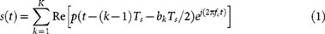where bk is the k-th transmitted bit information, namely,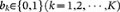and Ts is the symbol duration. Here, p(t) represents the baseband signal, which is given bywhere A and Tw are the amplitude of the baseband signal and the pulse width, respectively. Note that, in this paper, the bandwidth of the baseband signal fb = 1/Tw is much smaller than the carrier frequency fc of 4.4 GHz (narrow-band condition). From the experimental results in Fig. 3, we observed that the metasurfaces worked well with pulse widths longer than 0.01 μs. This means that the maximum bandwidth limit reaches only 100 MHz. Here this bandwidth is just about 2% of the carrier frequency used in the communication system. Therefore, even if we choose the maximum bandwidth of 100 MHz, the channel still satisfies the narrow-band condition. Consequently, even if we set several kinds of the pulse width (we set Tw to 20, 2000 and 20000 ns in this paper), the transmitted signal can be assumed as if it has only a single frequency component of the carrier frequency, namely, the three channels with 20-, 2000- and 20000-μs-long pulse widths can be assumed to have the same channel conditions. In addition, we use the same pulse interval for different pulse widths in order to fix the data speed at the same value.

Fig. 4 also shows the structure of the receiver. Here, we pay attention to the energy detection (non-coherent detection) as the received detection scheme. In the energy detection, since the binary PPM scheme chooses one from two location assignments in the k-th symbol, we calculate two kinds of k-th energies for the corresponding pulse locations from the received signal r(t) at the two kinds of time durations as follows: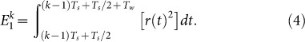Comparing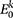with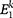, the received bit information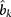can be decided as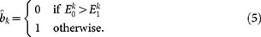As seen from this equation, the PPM requires no threshold. On the other hand, on-off keying (OOK) modulation, which is often used in digital communications with a pulse modulation scheme, requires a threshold to distinguish signals and noise. Because the threshold depends on the signal-to-noise power ratio (SNR), it is difficult to determine it in advance. In this sense, the PPM is superior to the OOK modulation. Furthermore, the symbol timing in Fig. 4 is synchronised with pilot signals.

Fig. 5 shows bit error rate (BER) performances using four-types of waveform-selective metasurfaces (Figs. 2(b) and (e) and the closed circles of Figs. 3(b) and (e), respectively, for Figs. 5(b), (c), (d) and (e)) together with transmitted powers calculated from TEM waveguide simulations as the received signals (i.e. as the blue line of Fig. 5(a)). These signals then experience the additive white Gaussian noise (AWGN) channel inside the demodulator (the red line). In Figs. 5(b) to (d) a signal becomes more erroneous due to the selective absorption of the metasurface, if the curve is shifted upwards from that without surface under test (SUT). For example, in Fig. 5(b), the 20-μs-long signal exhibited almost the same result as that without SUT, whereas the 0.02-μs-long signal showed reduced performance due to the waveform-selective metasurface. That is, in this case, the proposed waveform-selective communication can receive the signal with 20 μs pulse width and eliminate that with 0.02 μs pulse width. Note that even in these simulations our metasurfaces are not used as a band pass filter to eliminate low or high frequency components. From this perspective, it can be concluded that the waveform-selective wireless communication successfully receives wireless signals with arbitrary pulse widths even at the same carrier frequency.